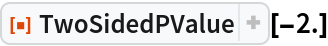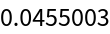Function Repository Resource:

# TwoSidedPValue

Find the probability of the absolute value of the test statistic being at least as extreme as the given value

Contributed by: Wolfram Research
 ResourceFunction["TwoSidedPValue"][x] gives the two-sided cumulative density beyond x for a normal distribution with zero mean and unit variance.

## Details and Options

The one-sided p-value is CDF[NormalDistribution[0,1],x] for x<0 and 1-CDF[NormalDistribution[0,1],x] for x0.
The two-sided p-value is twice the one-sided p-value.

## Examples

### Basic Examples (1)

The two-sided p-value for -2:

 In:=Out=## Requirements

Wolfram Language 11.3 (March 2018) or above

## Version History

• 1.0.0 – 03 May 2019